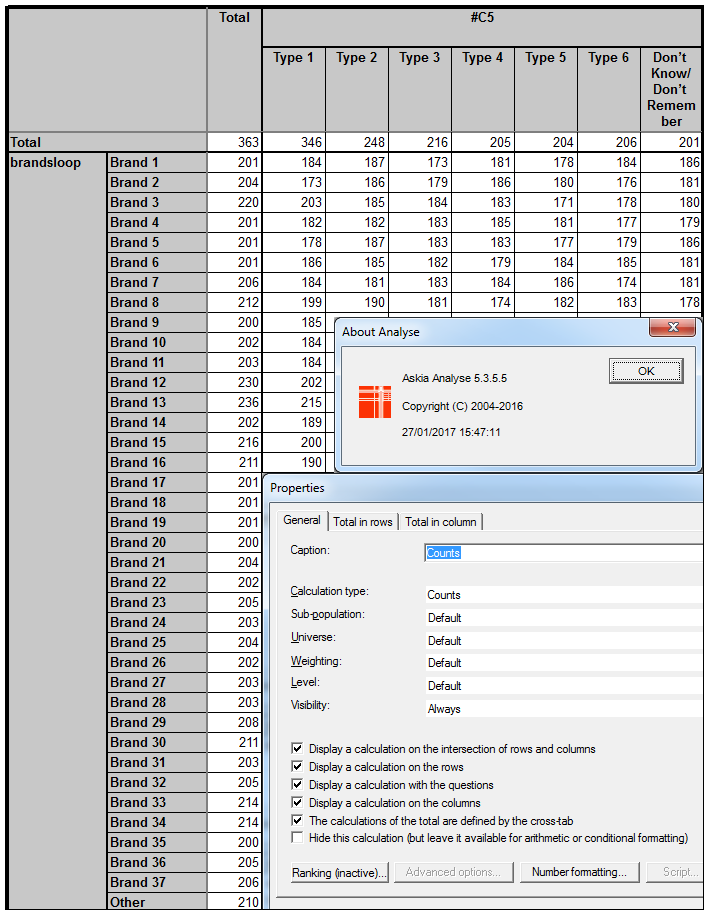Scroll

# Create Loops & Multiples by Script

Follow

 Summary This article introduces the 'Create Loop' functionality for transforming repeated questions in to a loop. It steps through examples of how to use the menus and scripts to achieve these calculated variables. to Askia Analyse. Written for Data Processors, Analysts, Researchers, Statistical Analysts. Keywords Closed by single script, Closed level, Open by script, Numeric by script, Calculated variable, NextQuestion, Loop, Superposed, Added table, Response level, Respondent level, askia Surf, Transform into loop, Transform into multiple, askia Tools, Scripts, Calculated responses, Multiple question, Numeric question, Aggregated script, Statistical calculations, ColSig, Calculated response, Mean, Median, Standard deviation, Run the totals in the sub-level, sub level.

Contents:

Why do we need to create loops in Analyse?

Sometimes you have repeated questions in your data file that have not been designed as a loop at the data collection stage or are so because they have been imported from another software package that does not maintain such a structure e.g. SPSS.

If you want to create a single table that adds the counts (changes level) at each of these variables then it gets a bit tricky without pushing these into a loop.

e.g.1 - Adding tables by calculated responses - you can create a variable in Analyse 'by profile' of the 2 or more tables you want to add. This joins the code-frames together. Then, in your table definition, add the counts of the categories together by using a calculated responses. They would have the syntax {1}+{11},  {2}+{12}, {3}+{13} and so on (for a 10 point scale). It could get even more tedious depending on how you want the % to show.

e.g.2 - Using table arithmetic to add values in cells - you can create some long and complicated scripts using table arithmetic to add counts and deal with the added percentages in different ways but this gets further complicated if there is a requirement to produce col sig test results or completely unmanageable if you need to start aggregating stat calculations like mean, median, standard deviation etc.

e.g.3 - Using askia tools to build multiples and loops - you can create multiples and loops easily using this functionality. However, you cannot create loops within loops without using askia design to write edits routings and then askia tools to edit the data into the desired structure. The loops can take a while to build if you have a lot of data and the steps have to be done in stages i.e. select all multiples,  run the build process, create all loops, run the build process etc. Finally, using askia tools to build loops has the main drawback that it's very time consuming to apply to Surf (.qew) set-ups with many wave .qes files.

The ease of having individual variables in a loop becomes apparent. You can switch between the respondent level (superposed tables) and the response level (added tables) simply by tabulating the looped variable and changing the 'Level' in your tab definition: e.g: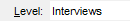to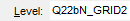The benefits of creating a multiple response set from several single variables are almost obvious; you can display your data with more flexibility and work out calculations such as mention rate and average number of responses etc. This is already possible in analyse so the main focus will be on the utility of creating loops.

How do I get started creating a loop?

The easiest method is to highlight your questions > Right click > Create loop. After this Analyse gives you the opportunity to name your loop and child question. It creates the questions in the calculated questions chapter.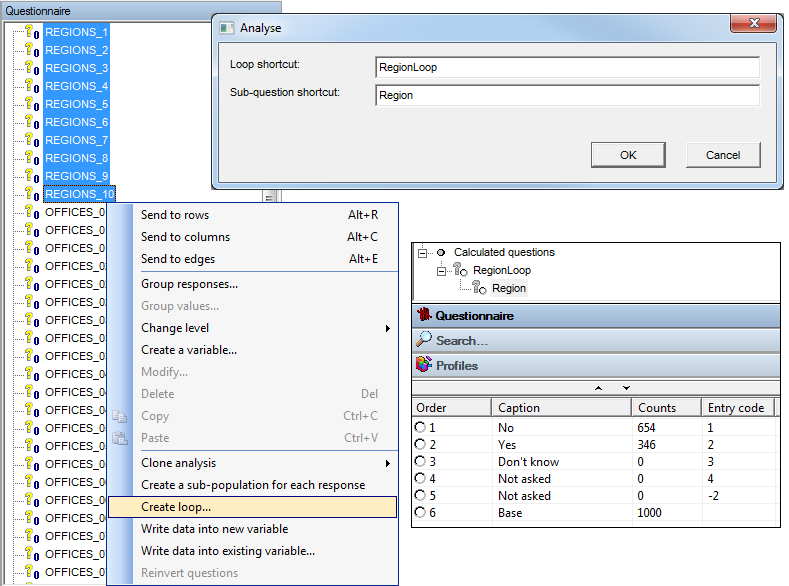The loop question is created as a 'Closed level' variable type. The script definition for the loop is auto-generated and can be seen below. In this case the script left empty is the same as the auto generated script because all respondents answered each of the 10 questions I created a loop from.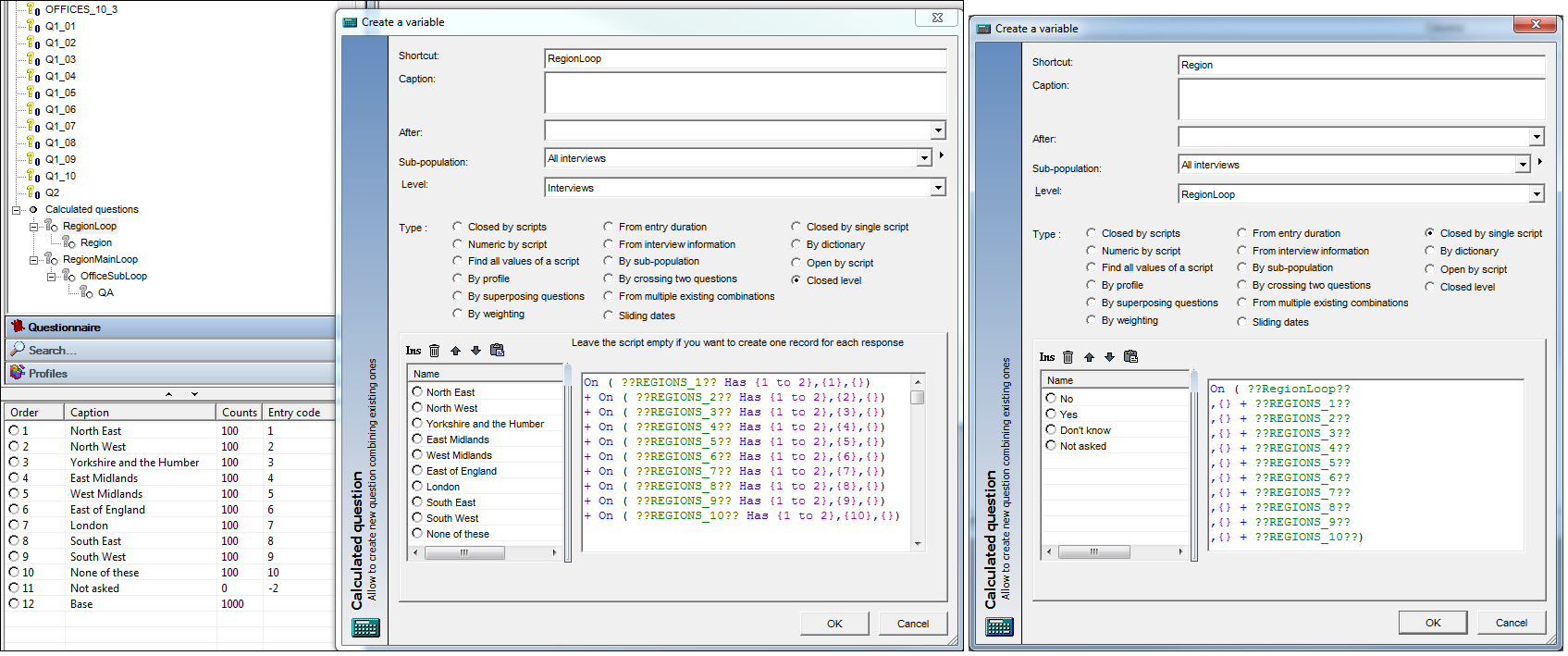The child question is created as a 'Closed by single script' variable type and again has its script auto generated. The useful point about this is that the script can be tweaked to exactly specify which respondents are shown in the loop.

See RegionLoop & Region variables in the attached TransExampleLoop.qes

How do I create a loop within a loop?

The flat list of questions below relates to the 10 regions, each with 3 office types and a No/Yes question for each of the 30 locations.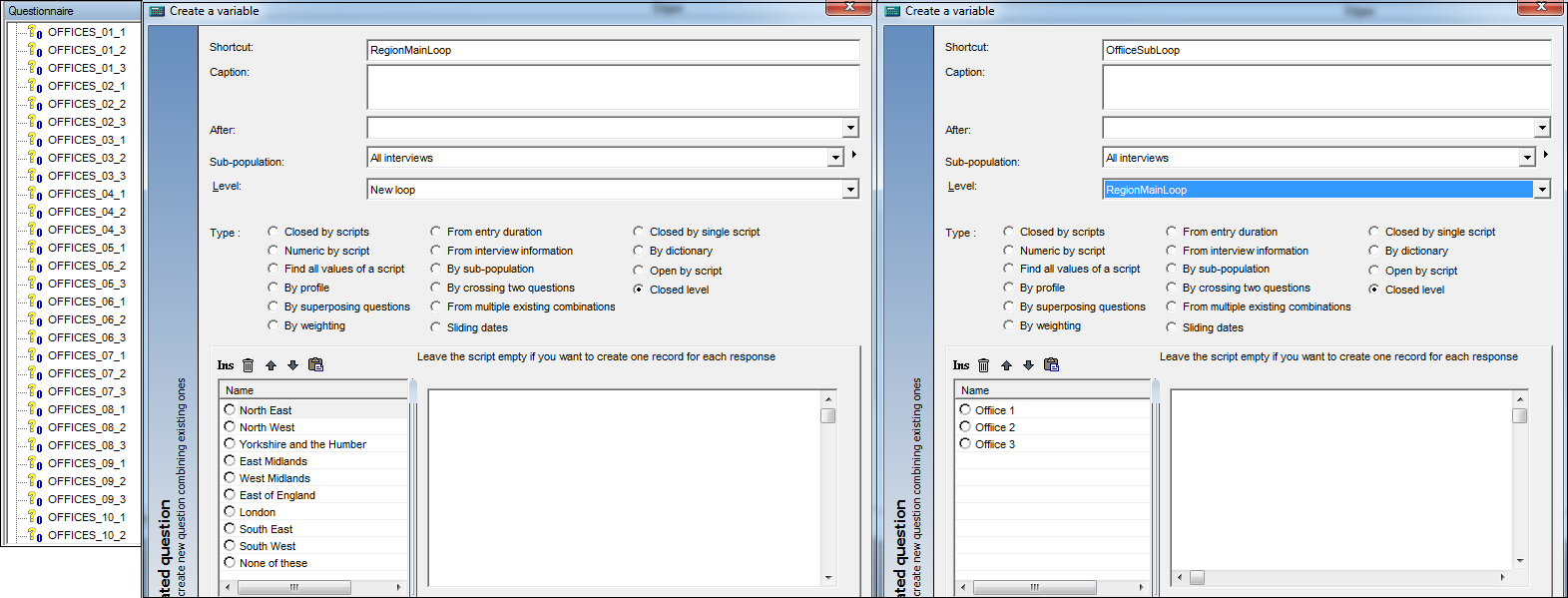First create a variable (closed level) called RegionMainLoop. Leave the definition empty for this simple example. Do the same again but this time name your closed level variable OffiiceSubLoop and set its level to be RegionMainLoop.

Now the last step is to create a variable type 'Closed by single script'. The script to apply in this particular case is: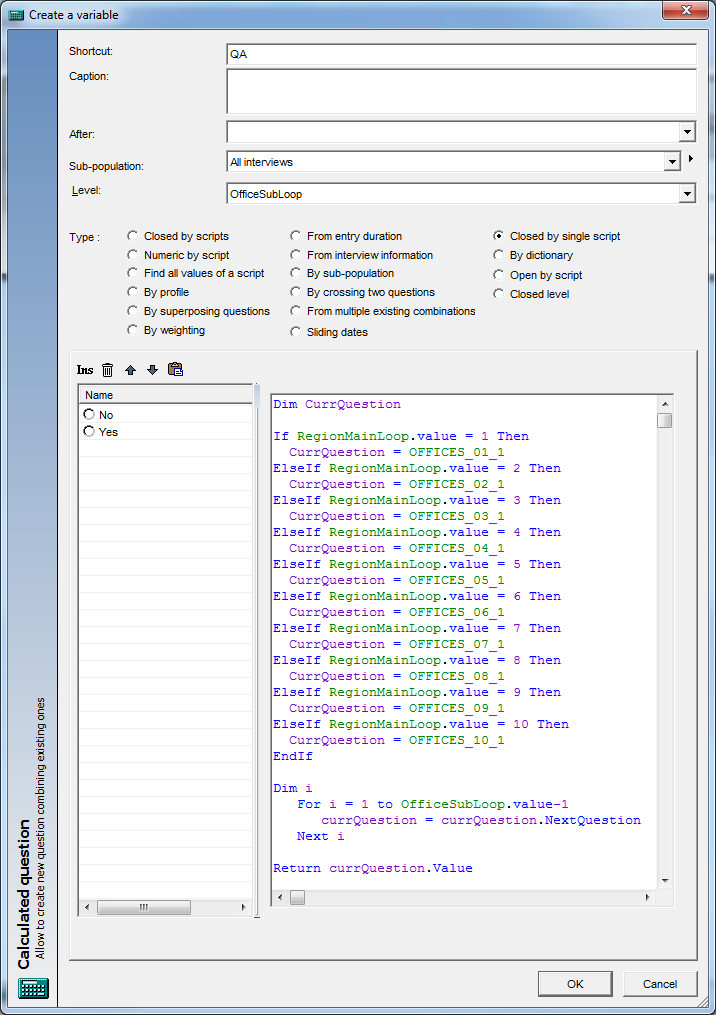The syntax above sets the correct question depending on what item of RegionMainLoop and OfficeSubLoop you are on.

The first section of syntax from ("If" up to "Dim i") places you at the first of three questions in the correct Region.

The section section of syntax from ("Dim i" up to "Return") places you at the correct one of three offices.

Let's take an example: RegionMainLoop = 8 and OfficeSubLoop = 3

RegionMainLoop.Value = 8 therefore you start at OFFICES_08_1

OfficeSubLoop.Value = 3 (and so OfficeSubLoop.Value-1 = 2) therefore you will loop through i and the syntax within the loop twice.

1st loop of i:   currQuestion = currQuestion.NextQuestion  =>
currQuestion = OFFICES_08_1.NextQuestion = OFFICES_08_2

2nd loop of i:   currQuestion = currQuestion.NextQuestion  =>
currQuestion = OFFICES_08_2.NextQuestion = OFFICES_08_3

It's worth noting that for i = 1 to 0 means you do not loop through i

To get used to creating loop within loops I have attached the .qes file relating to the above examples as well as another example shown below for this question structure.

Above: See RegionMainLoop, OfficeSubLoop & QA variables in the attached TransExampleLoop.qes

Below: See ML-ratSL-ratQ-rat variables in the attached MasterRatingExampleLoop.qes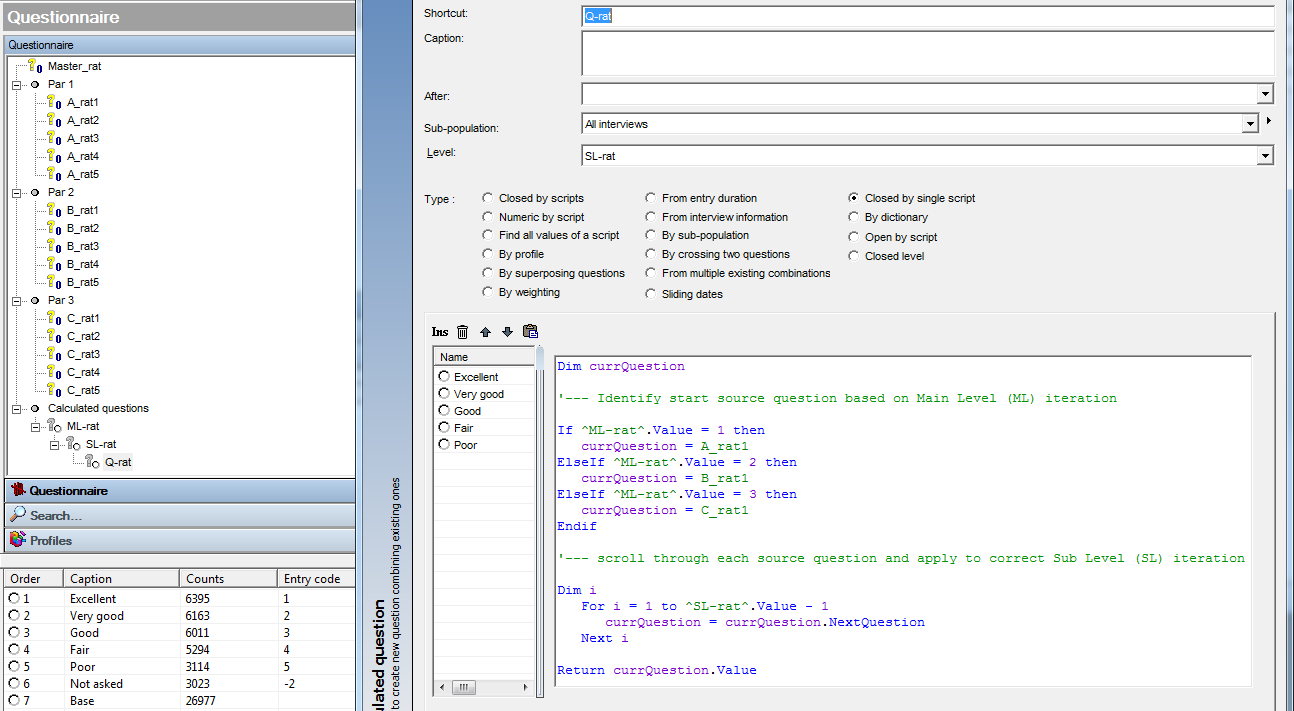How do I create a simple multiple question?

See #SM_BP1 variables in the attached BrandsExampleLoop.qes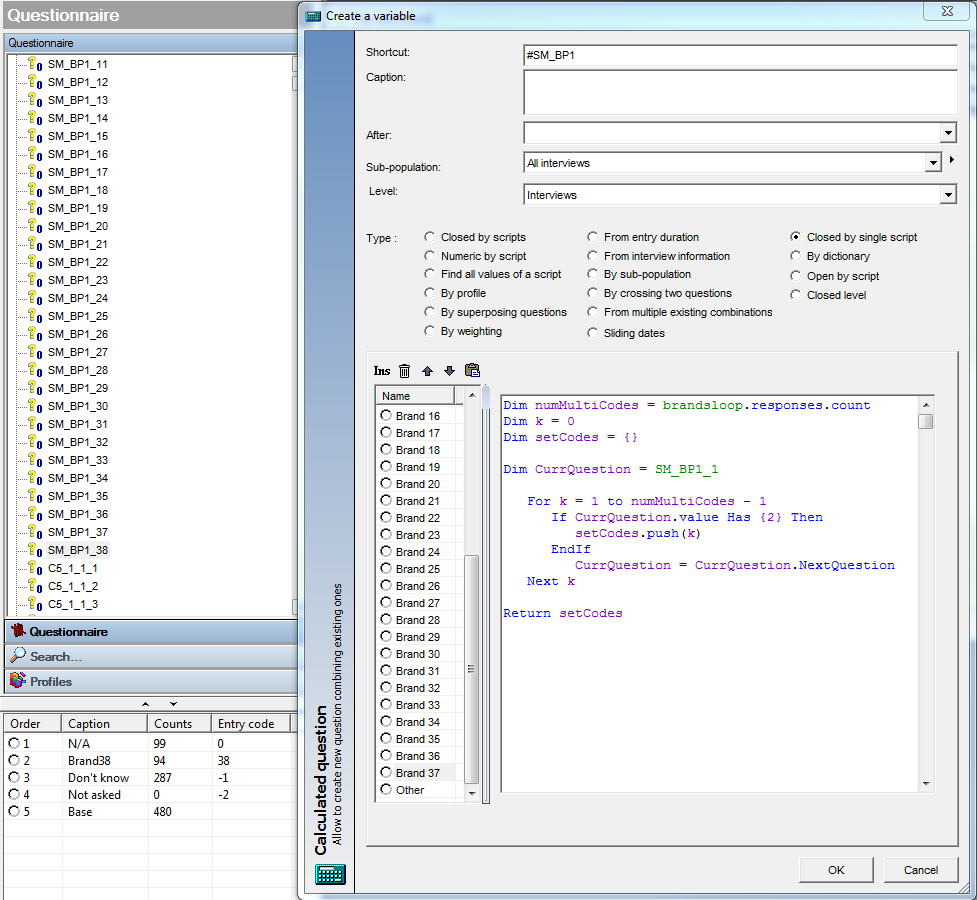We have 38 variables that we want to turn into a multicode, those selecting code 2 at SM_BP1_1 to SM_BP1_38. The idea here is that we define the start variable: SM_BP1_1

Then we iterate the current question 38 times in the loop k.

Each time we check if the current question has the value 2: - If CurrQuestion.value Has {2}

If it does, we fill an array (setCodes) with the iteration (code number) where the current question had a value of 2: - Then setCodes.push(k)

Then we return setCodes as our multiple response set.

How do I create a multiple question in a loop?

See brandsloop2 and #G2 variables in the same attached BrandsExampleLoop.qes

The scenario here is that we have batches of six questions G2_1_1 to G2_1_6 that are part of a multiple response set. These batches of six questions are repeated 38 times so the last six questions are: G2_38_1 to G2_38_6. This basically describes a six coded multiple in a 38 iteration loop.

For this we start by creating our closed level variable in the usual way: with blank script and the number of codes pasted in for the number of iterations we need.

Then we crate our 'Closed by single script' variable and set its level to be the closed level question we just created previously: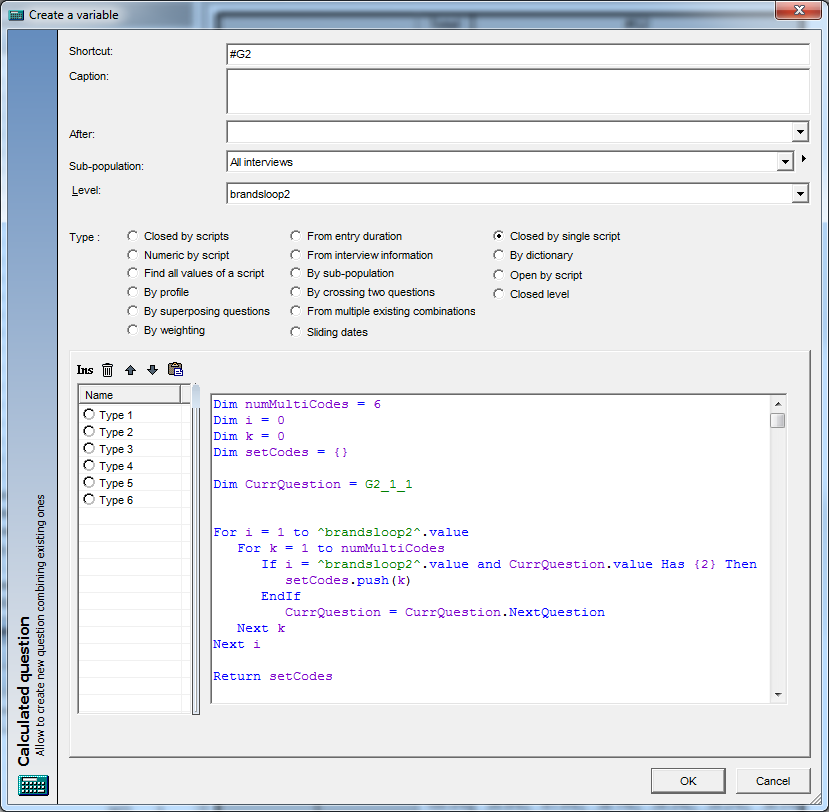We take the previous syntax (in the k loop) for a simple multiple and simply insert it in another loop (i loop) which iterates 38 times.

How do I create a multiple question in a loop within a loop?

See objectloopbrandsloop and #C5 variables in the same attached BrandsExampleLoop.qes

Here, the scenario is: 4 objects, 38 brands, 7 categories of the multi question:

• A batch of 38 x 7 question run C5_1_1_1 to C5_1_38_7
• A batch of 38 x 7 question run C5_2_1_1 to C5_2_38_7
• A batch of 38 x 7 question run C5_3_1_1 to C5_3_38_7
• A batch of 38 x 7 question run C5_4_1_1 to C5_4_38_7

Again, this follows on from the previous example. You keep the structure of your syntax the same apart from the definition of your current question. In the case of a loop within a loop, your 'CurrQuestion' start position needs to be defined for each code of the outerloop: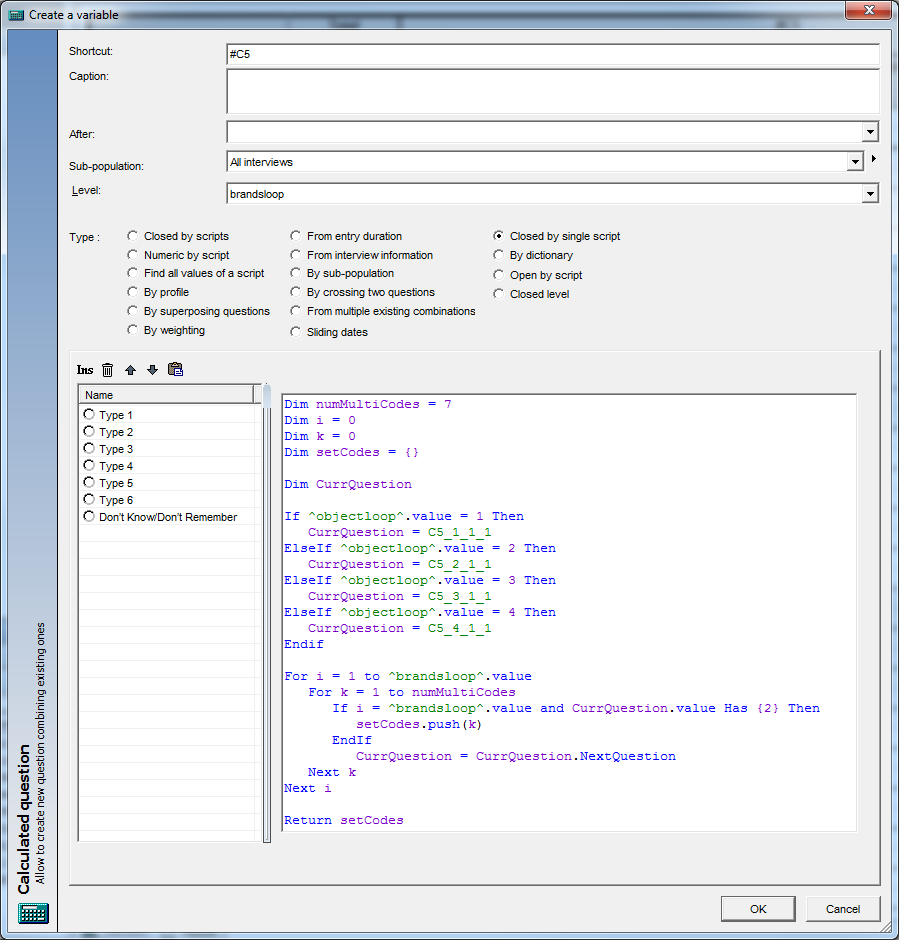Whilst we're on the subject of loops within loops, take a look at variable #D8. This shows how to put together a numeric question within two loop levels.

One last point is to explain when you might need to put a script in a 'Closed level' variable type. In brandsloop in the example above it's left empty but what does this mean in terms of the data we see?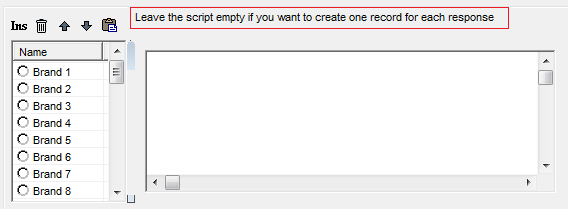If you leave it empty, Analyse will act as if a respondent has been through each iteration of the loop. You can see the outcome of this boxed in red for the total column of a simple cross tab, brandsloop x #C5.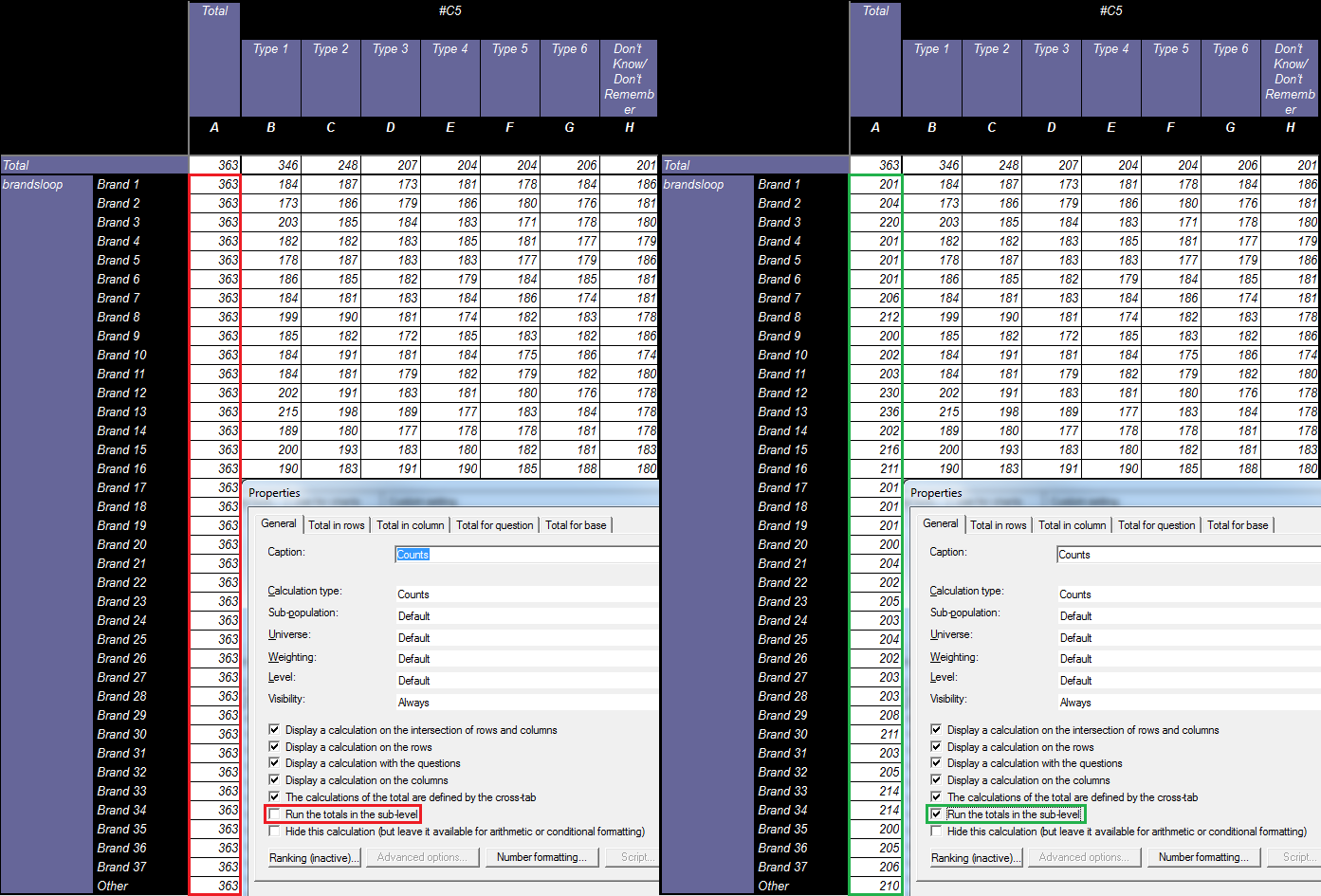This is what you will see in versions of Analyse less than 5.4.5.0. Since that version, however, we have added an option to all calculations that will create a total for you based on those answering the sub question, not from who has seen each iteration of the parent level. This option is shown above: 'Run the totals in the sub-level'.

If you want to achieve the results boxed in green above in a version less than 5.4.5.0, you will need to write some script. The quick way to do this is to take the script from you child question #C5 and paste it into the script window for brandsloop. In there, simply change setcodes.push(k) to be setcodes.push(i).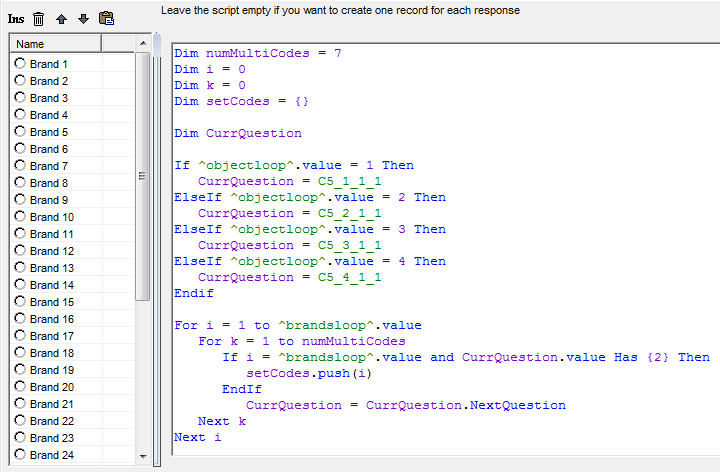The result: